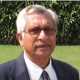## About Suresh Goel

Alliance World School ( Director ) A Level Maths / AP Calculus Teacher Former Principal : Air Force School, Lodhi Road, New Delhi

# AS-LEVEL FURTHER MATHEMATICS (9231) – POLAR COORDINATESGlimpse of AS Level Further Maths – Polar Coordinates Notes

Related Content

# AS-LEVEL FURTHER MATHEMATICS (9231) – VECTOR IN 3DGlimpse of AS Level Further Maths – Vector in 3D Notes

Related Content

# AS-LEVEL FURTHER MATHEMATICS (9231) – MATRICESGlimpse of AS Level Further Maths – Matrices Notes

Related Content

# AS-LEVEL FURTHER MATHEMATICS (9231) – SUMMATION OF SERIESGlimpse of AS Level Further Maths – Summation of Series Notes

Related Content

# AS-LEVEL FURTHER MATHEMATICS (9231) – ROOTS OF POLYNOMIALSGlimpse of AS Level Further Maths -Roots of Polynomials Notes

Related Content

# AS-LEVEL FURTHER MATHEMATICS (9231) – PROOF BY INDUCTIONGlimpse of AS Level Further Maths -Proof by Induction Notes

Related Content

# A-LEVEL MATHEMATICS (9709) – DIFFERENTIAL EQUATIONSGlimpse of A Level Maths -Differential Equations Notes

Related Content

# A-LEVEL MATHEMATICS (9709) – INTEGRATION BY SUBSTITUTION AND BY PARTSGlimpse of A Level Maths – Integration by Substitution and Integration By Parts Notes

Related Content

# A-LEVEL MATHEMATICS (9709) – COMPLEX NUMBERSGlimpse of A Level Maths – Complex Numbers Notes

Related Content